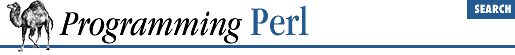home | O'Reilly's CD bookshelfs | FreeBSD | Linux | Cisco | Cisco Exam

#### 3.2.156 sprintf

```sprintf ```

FORMAT
```
, ```

LIST
```
```

This function returns a string formatted by the usual printf conventions. The ``` FORMAT ``` string contains text with embedded field specifiers into which the elements of ``` LIST ``` are substituted, one per field. Field specifiers are roughly of the form:

```%```

m.nx
```
```

where the ``` m ``` and ``` n ``` are optional sizes whose interpretation depends on the type of field, and ``` x ``` is one of:

Code Meaning
``` c``` Character
``` d``` Decimal integer
``` e``` Exponential format floating-point number
``` f``` Fixed point format floating-point number
``` g``` Compact format floating-point number
``` ld``` Long decimal integer
``` lo``` Long octal integer
``` lu``` Long unsigned decimal integer
``` lx``` Long hexadecimal integer
``` o``` Octal integer
``` s``` String
``` u``` Unsigned decimal integer
``` x``` Hexadecimal integer
``` X``` Hexadecimal integer with upper-case letters

The various combinations are fully documented in the manpage for printf(3) , but we'll mention that ``` m ``` is typically the minimum length of the field (negative for left justified), and ``` n ``` is precision for exponential formats and the maximum length for other formats. Padding is typically done with spaces for strings and zeroes for numbers. The ``` *``` character as a length specifier is not supported. But, you can easily get around this by including the length expression directly into ``` FORMAT ``` , as in:

```\$width = 20; \$value = sin 1.0;
foreach \$precision (0..(\$width-2)) {
\$output_arr[\$precision] = sprintf "%\${width}.\${precision}f", \$value;
}```